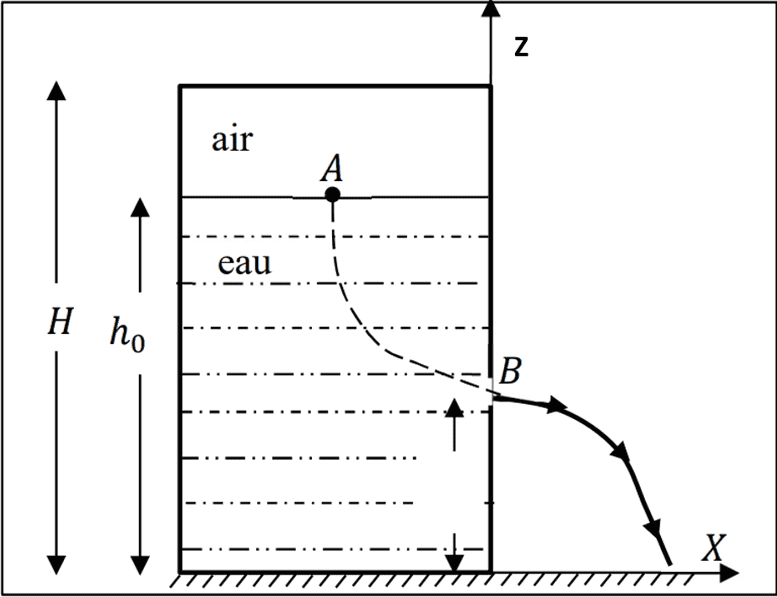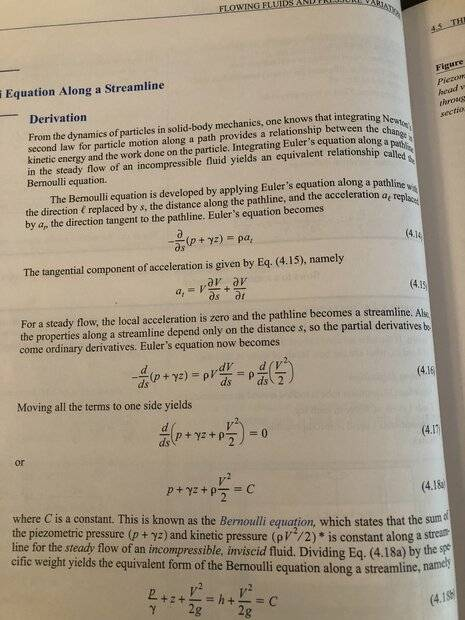# Calculating flow rate when emptying liquid from a closed-top tank

• labuch
P_{atm}=\frac{P_1}{\gamma}+z_1P_{atm}=\frac{P_1}{\gamma}+\frac{P_{atm}}{2g}\frac{z_1}{\gamma}P_{atm}=\frac{P_1}{\gamma}+\frac{P_{atm}}{2g}\frac{z_1}{\gamma}P_{atm}=\frac{P_1}{\gamma}+\frac{P_{atf

#### labuch

Homework Statement
Hello,
Here is my problem: "We have a closed tank of large size which contains a liquid topped by air at a pressure equal to the atmospheric pressure. If the tank is closed, as the water flows out, the volume of air above the free surface increases, so the pressure decreases (law of perfect gases). We seek to find the pressure variation (𝛥P) at the surface of the water in the tank as a function of the z axis (whose origin is at B) and to give the expression of v (the flow velocity) as a function of z."
Relevant Equations
I know that:
-according to the perfect gas equation P=nRT/V so that pressure is proportional to volume, so that P(t=0)*V(t=0)=P(t=1)*V(t=1)
-That the initial pressure at the surface of the water is P =Patm
-by applying bernoulli between A and B, we have classical V0=sqrt(2gh)
I can imagine the experiment: the pressure at the surface will drop while the tank is emptying (if no air bubbles enter through the evacuation of course, otherwise it restores the pressure atm at the surface). The flow speed decreases as a function of time until the external pressure maintains a liquid level above the drain (thus stopping the flow). I can't put this resonance in mathematical form (𝛥P as a function of z and v as a function of z)

thank you in advance for those who will help me,
Sincerely

here is a simple visualisation of the problemeHello @labuch ,##\qquad## !​

-by applying bernoulli between A and B, we have classical V0=sqrt(2gh)
No, you don't. That expression is based on pressure terms canceling, and in your case they don't.

But you can build an appropriate model along the same lines and end up with a differential equation. I don't expect that to have an analytical solution, but perhaps I can be proven wrong.
Interesting problem; not homework I suppose ?

##\ ##

•Lnewqban and labuch
Hello @labuch ,##\qquad## !​

No, you don't. That expression is based on pressure terms canceling, and in your case they don't.

But you can build an appropriate model along the same lines and end up with a differential equation. I don't expect that to have an analytical solution, but perhaps I can be proven wrong.
Interesting problem; not homework I suppose ?

##\ ##
hello, thank you for your response, Yes you are right I thought that this expression could be true at the very beginning of the draining that's why I called it V0 and I had the feeling that it was there that I had to dig. This homework is actually a problem around which I have to create a practical work. My problem is that I don't know how to develop the expression to solve the problem mathematically

here is a simple visualisation of the problemeView attachment 325111
Bernoulli's is not valid for this problem as the flow is not steady (its time varying).

I propose to begin with the "Momentum Equation" (Reynolds Transport Theorem - Newtons Second for a control volume) to develop your differential equation

$$\sum \boldsymbol F = \frac{d}{dt}\int_{cv} \rho \boldsymbol v ~dV\llap{-} + \int_{cs} \rho \boldsymbol v \boldsymbol V \cdot d \boldsymbol A$$

Although its application is probably going to be cumbersome and not obvious... the answer is going to be in there somewhereLast edited:
Bernoulli's is not valid for this problem as the flow is not steady (its time varying).

I propose to begin with the "Momentum Equation" (Reynolds Transport Theorem - Newtons Second for a control volume) to develop your differential equation

$$\sum \boldsymbol F = \frac{d}{dt}\int_{cv} \rho \boldsymbol v ~dV\llap{-} + \int_{cs} \rho \boldsymbol v \boldsymbol V \cdot d \boldsymbol A$$

Although its application is probably going to be cumbersome and not obvious... the answer is going to be in there somewhereHello,
I thought of another solution because I could not use your formula. I think that we can probably assimilate the tank to a tank of right section of constant area S. We can note z=f(t) the height of air in the tank and Zo the initial value. The law of perfect gases applied by considering the temperature as fixed leads to :
Patm.zo=P.z
The velocity of the fluid at the upper free surface of the liquid is (dz/dt).
If we note "s" the cross-sectional area of the outlet orifice, the conservation of flow gives: S.(dz/dt)=s.Vs where Vs is the outlet velocity at the bottom of the tank. Taking into account these two equations, Bernoulli's theorem will give you the differential equation verified by z=f(t). However I can't get to the final equation...

Bernoulli's is not supposed to be valid for non-steady flow, but then again in my fluids text does use the solution ## v = \sqrt{2gh}## for the exit velocity in a draining tank example problem...like we find in Torricelli's Law derived from Bernoulli's principle (so I'm not sure what exactly is affected and to what extent under this assumption).

Under that assumption (which also neglects viscosity), let 1 be the upper surface of the fluid, and 2 be the position of the fluid jet.

$$\frac{P_1}{\gamma} + \frac{v_1^2}{2g} + z_1 = \frac{P_2}{\gamma} + \frac{v_2^2}{2g} + z_2$$

##z_2## is the datum
##P_2## is 0 gage pressure (atmospheric)
## v_1 \ll v_2## the kinetic head in the tank is negligible in comparison to the jet at 2.
##A_1## is the cross section of the tank
##A_2## is the cross section of the jet
##H## is from ##z_2## to the top of the tank
##z_{1_o}## is the initial height of fluid in the tank measured from ##z_2##

$$\frac{P_1}{\gamma} + z_1 = \frac{v_2^2}{2g}$$

Let the absolute pressure above the tank surface 1 be ##P(z_1) = P_1(z_1) + P_{atm}##

Applying the ideal gas law we are looking for the pressure of the gas as a function of ##z_1##

$$P_{atm} A_1 ( H - z_{1_o}) = P(z_1)A_1( H - z_1)$$

Can you take it from there?

##P_1## will be a function of ##z_1##, and ##v_2## will be a function of ##\dot z_1##.

Last edited:
•ChiralSuperfields
While we are on the subject can anyone explain the consequences of applying Bernoulli's to non-steady flow?

Here is the derivation in my textbook. The local acceleration (i.e. the variation of velocity with time at a specific point on a path line - ## \frac{\partial V}{\partial t}## in this problem will clearly not be zero, which negates everything after (4.15).•ChiralSuperfields
Based on the figure in post #2, here is my take on this.

Ideal Gas Law: $$P_g(H-h)=P_{atm}(H-h_0)$$where ##P_g## is the absolute pressure of the gas in the head space.

Bernoulli Equation: $$P_g+\rho g h=P_{atm}+\rho g h_B+\frac{1}{2}\rho v^2$$

Combining these two equations gives: $$\frac{1}{2}\rho v^2=\rho g(h-h_B)-\left(\frac{h_0-h}{H-h}\right)p_{atm}$$

Finally, the overall mass balance is $$A_{tank}\frac{dh}{dt}=-A_{exit}v$$

••ChiralSuperfields, BvU, erobz and 1 other person
I found this example of Unsteady Bernoulli's. Can someone reconstruct an interpretation from the example? There is obviously a change in the flow energy per unit volume (a deviation from Bernoulli's for steady flow), but where/what is it?

••erobz and berkeman